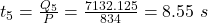How much total heat transfer is necessary to lower the temperature of 0.175 kg of steam from 125.5 °C to −19.5 °C, including the energy for

Question

How much total heat transfer is necessary to lower the temperature of 0.175 kg of steam from 125.5 °C to −19.5 °C, including the energy for phase changes? How much time is required for each stage of this process, assuming a constant 835.0 W rate of heat exchange? Give the times in the order that the stages occur.

in progress 0
6 months 2021-07-28T15:06:32+00:00 1 Answers 6 views 0

Explanation:

The heat required to change the temperature of  steam from 125.5  °C to 100 °C is: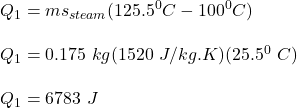The heat required to change the steam at 100°C to water at 100°C is;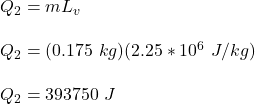The heat required to change the temperature from 100°C to 0°C is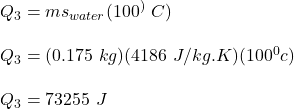The heat required to change the water at 0°C to ice at 0°C  is: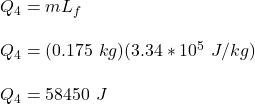The heat required to change the temperature of ice from 0°Cto -19.5°C is: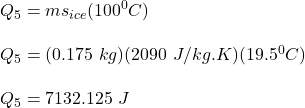The total heat required to change the steam into ice is:b)

The time taken to convert steam from 125 °C to 100°C is: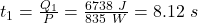The time taken to convert steam at  100°C to water at  100°C is: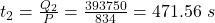The time taken to convert water to 100° C to 0° C is: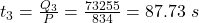The time taken to convert water at 0° to ice at 0° C is :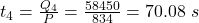The time taken to convert ice from 0° C to -19.5° C is: